Ex 9.2

Chapter 9 Class 11 Sequences and Series
Serial order wise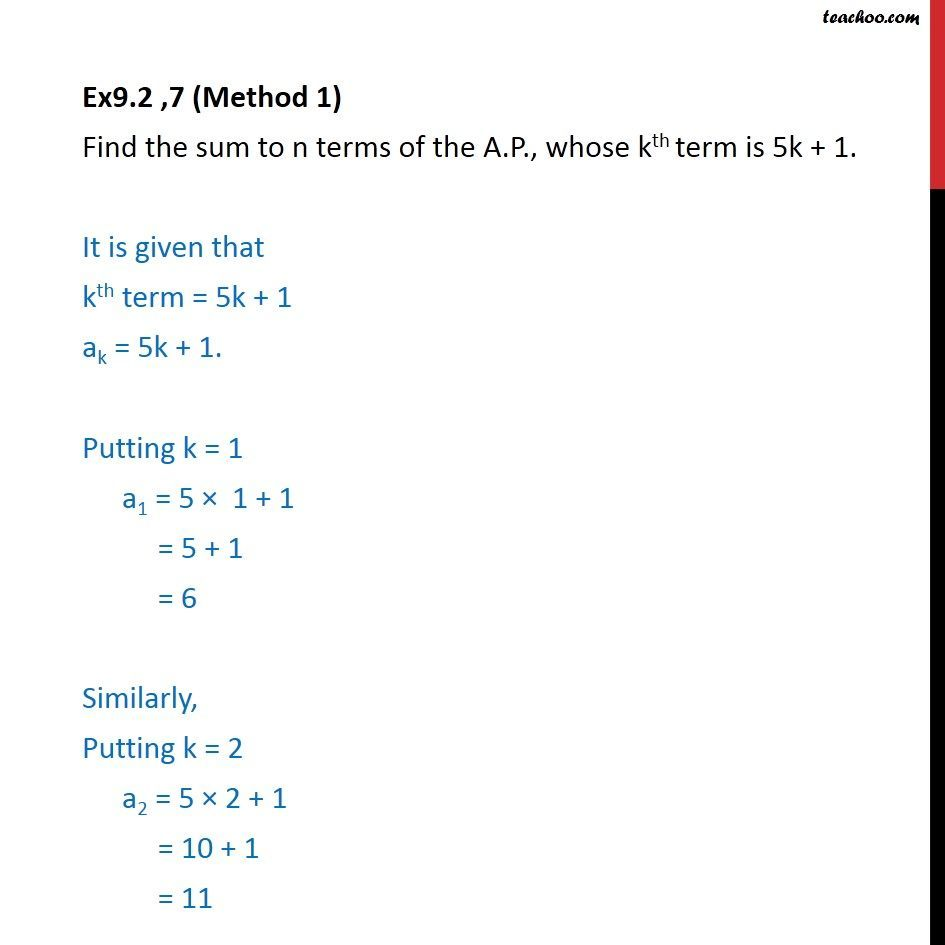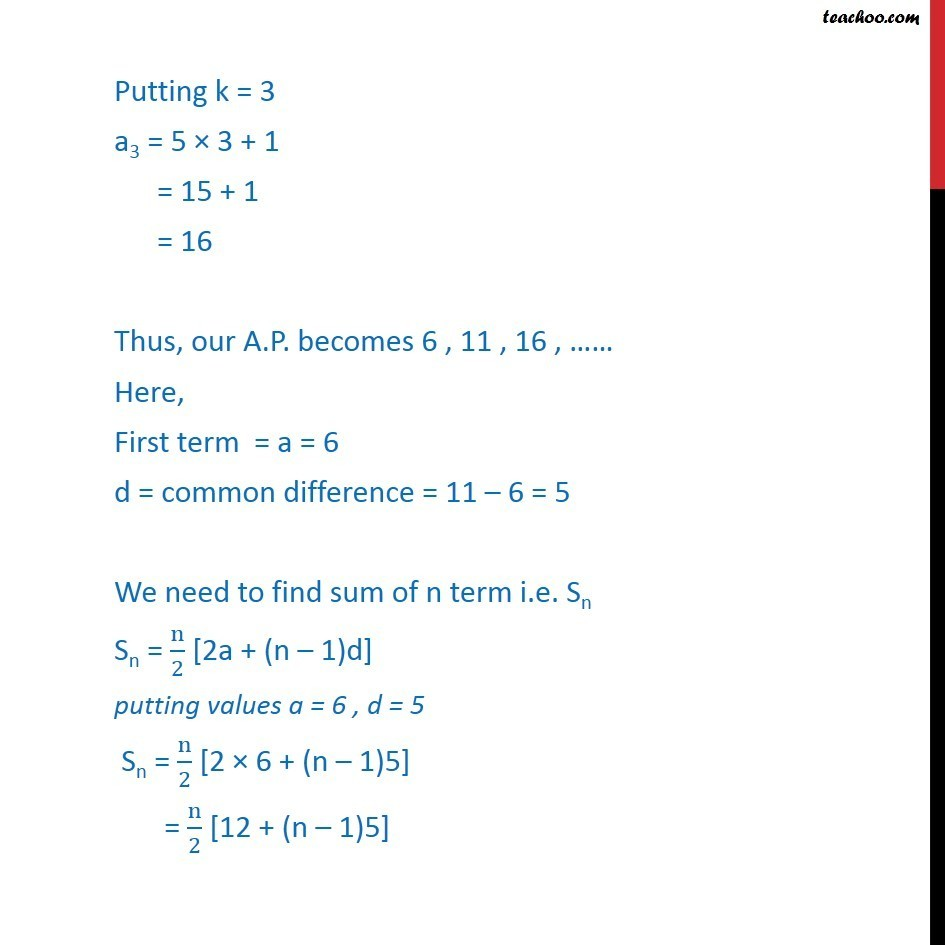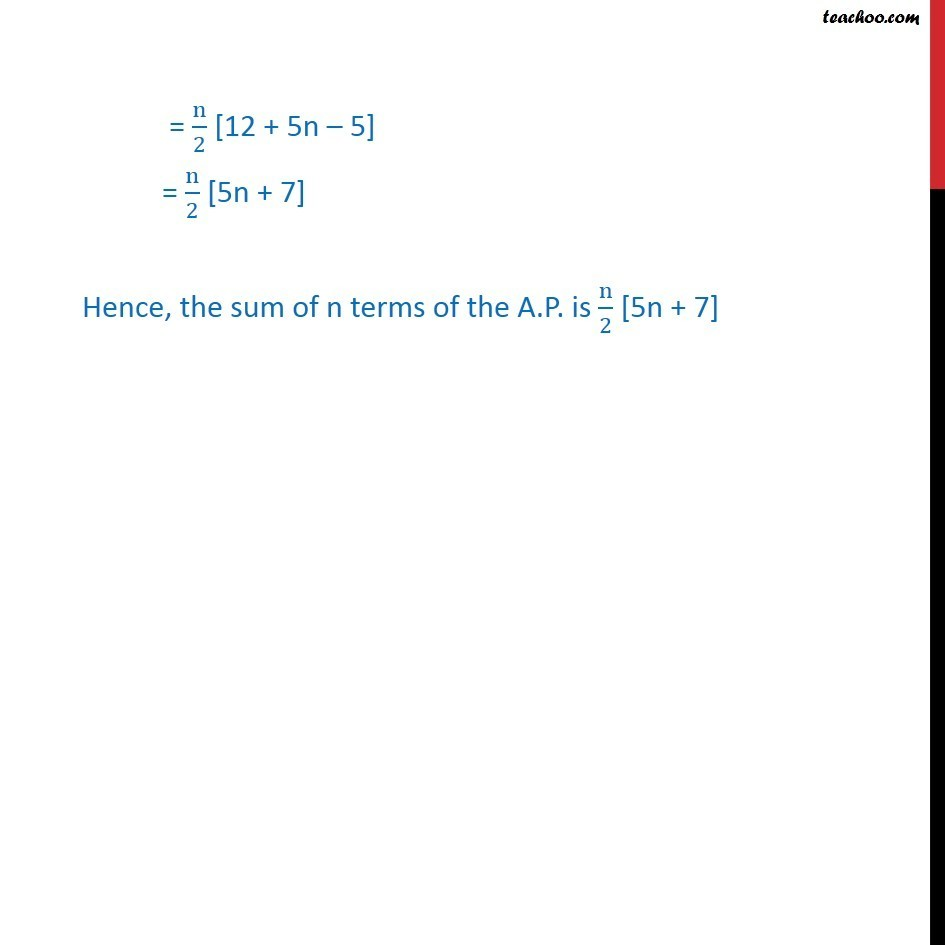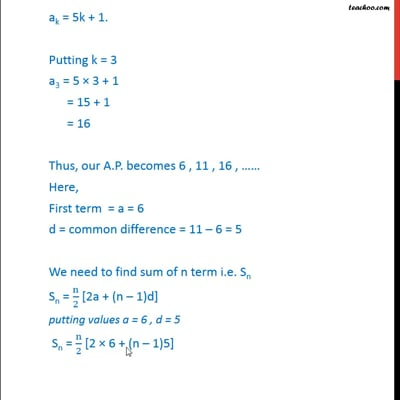This video is only available for Teachoo black users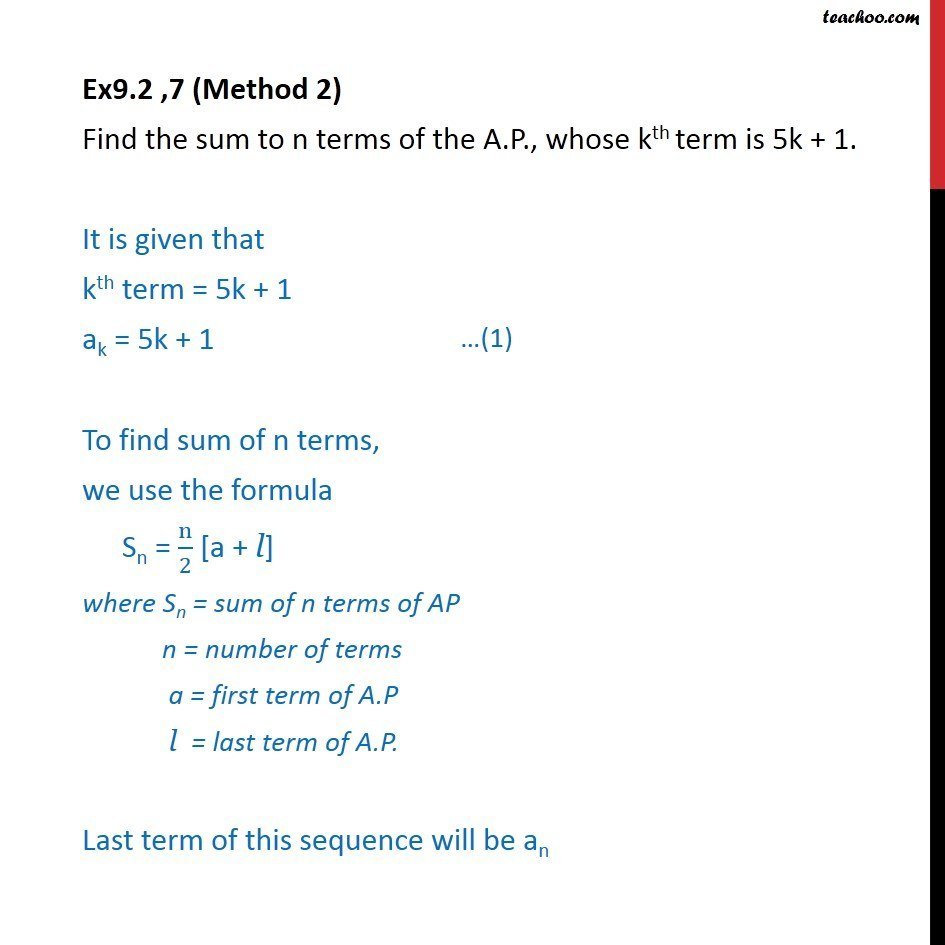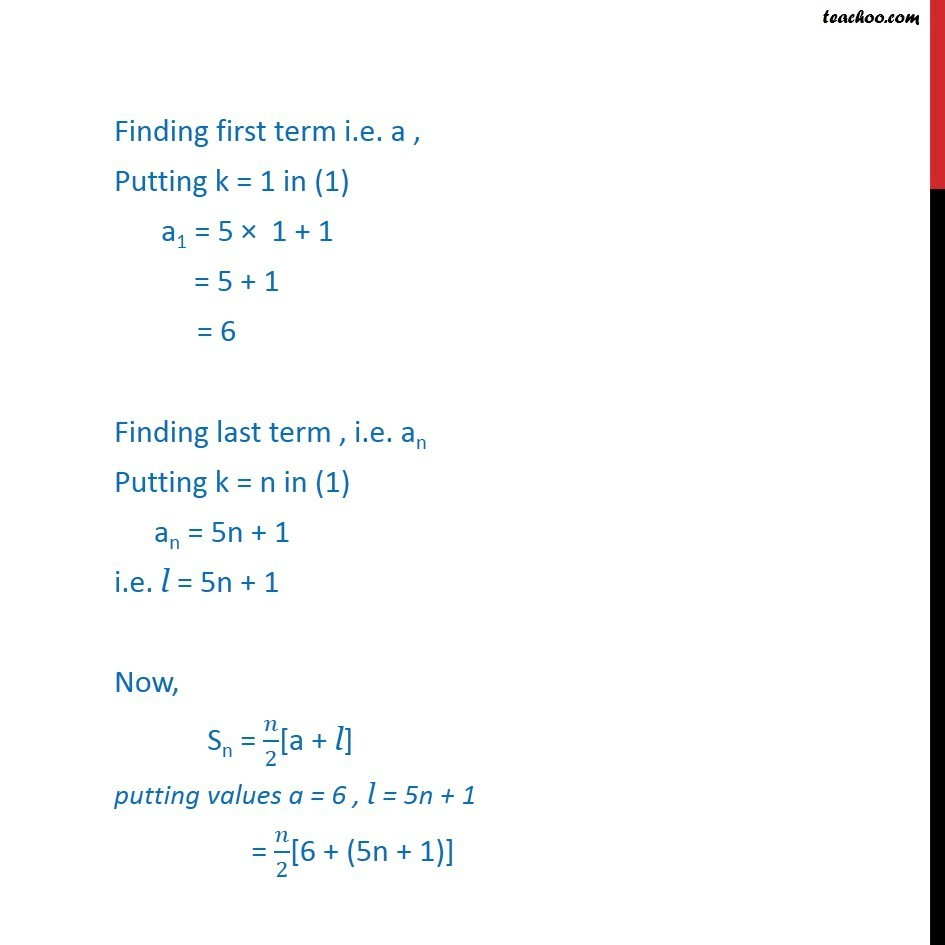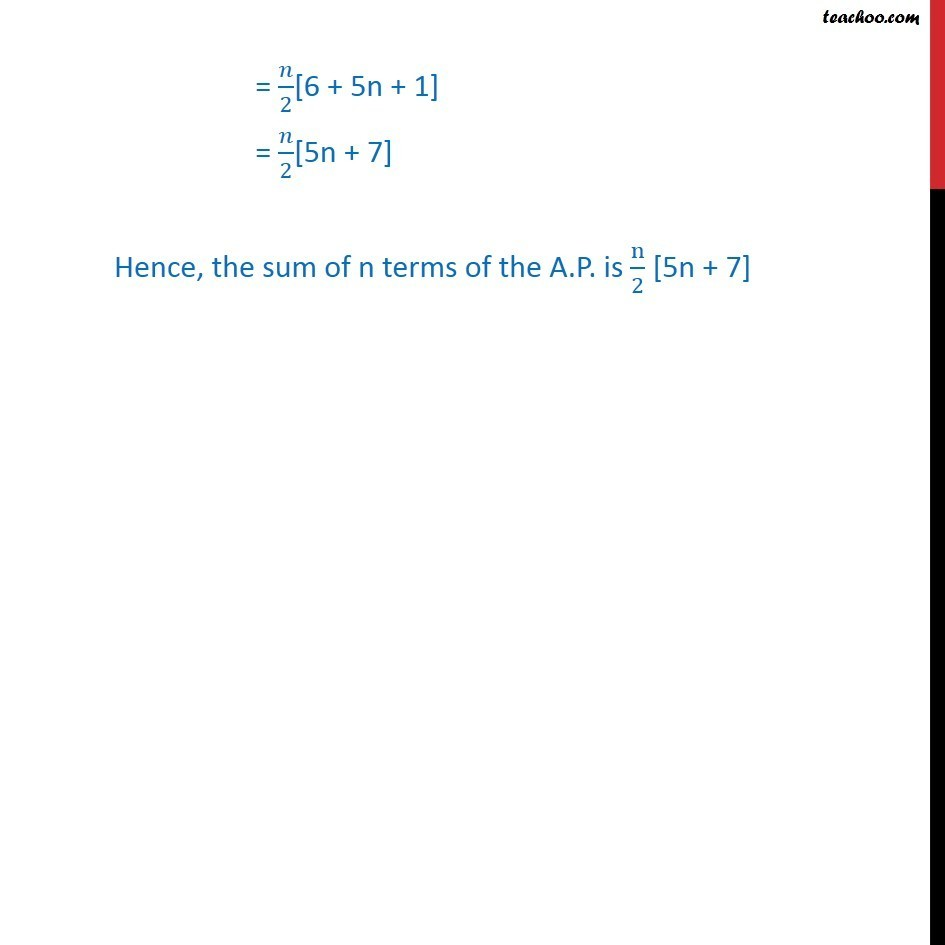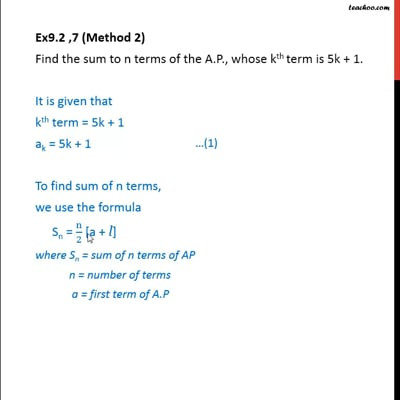This video is only available for Teachoo black users

Solve all your doubts with Teachoo Black (new monthly pack available now!)

### Transcript

Ex9.2 ,7 (Method 1) Find the sum to n terms of the A.P., whose kth term is 5k + 1. It is given that kth term = 5k + 1 ak = 5k + 1. Putting k = 1 a1 = 5 × 1 + 1 = 5 + 1 = 6 Similarly, Putting k = 2 a2 = 5 × 2 + 1 = 10 + 1 = 11 Putting k = 3 a3 = 5 × 3 + 1 = 15 + 1 = 16 Thus, our A.P. becomes 6 , 11 , 16 , …… Here, First term = a = 6 d = common difference = 11 – 6 = 5 We need to find sum of n term i.e. Sn Sn = n/2 [2a + (n – 1)d] putting values a = 6 , d = 5 Sn = n/2 [2 × 6 + (n – 1)5] = n/2 [12 + (n – 1)5] = n/2 [12 + 5n – 5] = n/2 [5n + 7] Hence, the sum of n terms of the A.P. is n/2 [5n + 7] Ex9.2 ,7 (Method 2) Find the sum to n terms of the A.P., whose kth term is 5k + 1. It is given that kth term = 5k + 1 ak = 5k + 1 To find sum of n terms, we use the formula Sn = n/2 [a + l] where Sn = sum of n terms of AP n = number of terms a = first term of A.P l = last term of A.P. Last term of this sequence will be an Finding first term i.e. a , Putting k = 1 in (1) a1 = 5 × 1 + 1 = 5 + 1 = 6 Finding last term , i.e. an Putting k = n in (1) an = 5n + 1 i.e. l = 5n + 1 Now, Sn = 𝑛/2[a + l] putting values a = 6 , l = 5n + 1 = 𝑛/2[6 + (5n + 1)] = 𝑛/2[6 + 5n + 1] = 𝑛/2[5n + 7] Hence, the sum of n terms of the A.P. is n/2 [5n + 7]